Next: Worked example 4.2: Block Up: Newton's laws of motion Previous: Frames of reference

## Worked example 4.1: In equilibrium

Question: Consider the diagram. If the system is in equilibrium, and the tension in string 2 is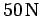, determine the mass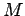.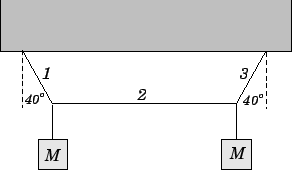Answer: It follows from symmetry that the tensions in strings 1 and 3 are equal. Let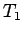be the tension in string 1, and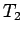the tension in string 2. Consider the equilibrium of the knot above the leftmost mass. As shown below, this knot is subject to three forces: the downward force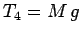due to the tension in the string which directly supports the leftmost mass, the rightward forcedue to the tension in string 2, and the upward and leftward forcedue to the tension in string 1. The resultant of all these forces must be zero, otherwise the system would not be in equilibrium. Resolving in the horizontal direction (with rightward forces positive), we obtain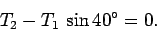Likewise, resolving in the vertical direction (with upward forces positive) yields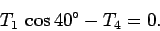Combining the above two expressions, making use of the fact that, gives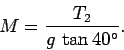Finally, since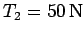and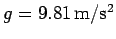, we obtain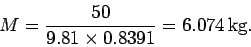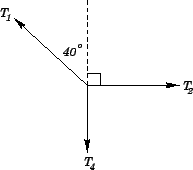Next: Worked example 4.2: Block Up: Newton's laws of motion Previous: Frames of reference
Richard Fitzpatrick 2006-02-02